# Math in Focus Grade 3 Chapter 6 Practice 4 Answer Key Multiply by 8

Go through the Math in Focus Grade 3 Workbook Answer Key Chapter 6 Practice 4 Multiply by 8 to finish your assignments.

## Math in Focus Grade 3 Chapter 6 Practice 4 Answer Key Multiply by 8

Complete the multiplication fact. Then show on the number line.

Question 1.
3 × 8 = ____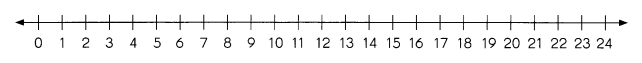3 × 8 = 24.

Explanation:
Here we have represented the number line of 3 × 8 which is 24, as it has 3 jumps of 8.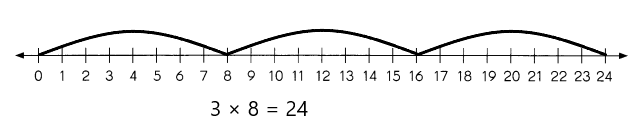Complete the multiplication fact. Then shade to show on the area model.

Question 2.
6 × 8 = ____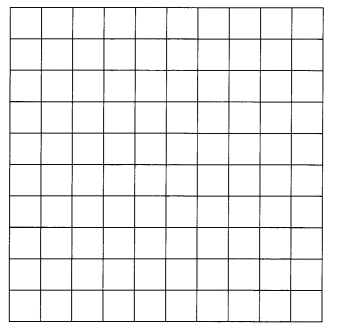6 × 8 = 48.

Explanation:
The model of 6 × 8  is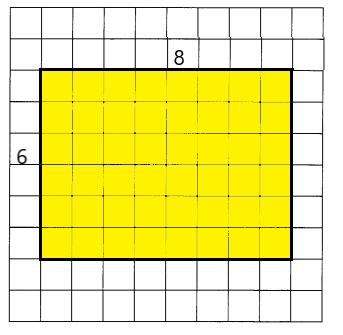Fill in the missing numbers.

Question 3.
8 eights = 8 × ___
8 eights = 8 × 8 64.

Explanation:
Given that 8 eights which means 8 × 8 are 64.
So the missing digit is 8.

Question 4.
3 eights = 8 × ____
3 eights = 8 × 3 = 24.

Explanation:
Given that 3 eights which means 3 × 8 are 24.
So the missing digit is 3.

Question 5.
6 + 6 + 6 + 6 + 6 + 6 + 6 + 6 = 8 × ____
6 + 6 + 6 + 6 + 6 + 6 + 6 + 6 = 8 × 6 = 48.

Explanation:
Given that 6 + 6 + 6 + 6 + 6 + 6 + 6 + 6, and we can write this as 8 × 6 which is 64.
So the missing digit is 6.

Question 6.
8 + 8 + 8 + 8 + 8 + 8 = 6 × ____
8 + 8 + 8 + 8 + 8 + 8 = 6 × 8 = 48.

Explanation:
Given that 8 + 8 + 8 + 8 + 8 + 8, and we can write this as 6 × 8 which is 48.
So the missing digit is 8.

Question 7.
5 × 8 = 8 × ____
5 × 8 = 8 × 5 = 40.

Explanation:
Here, by using commutative property we can write 5 × 8 = 8 × 5 which is 40.

Question 8.
5 eights = 8 + 8 + ___ + ___ + ____
5 eights = 8 + 8 + 8 + 8 + 8 = 40

Explanation:
Given that 5 eights which means 5 × 8 are 40.
So 8 + 8 + 8 + 8 + 8 = 40.

Multiply. Use multiplication facts you know to find other multiplication facts.

Question 9.
8 × 4 = 10 groups of 4 – ___ groups of 4
= ___ – ____
= ____
8 × 4 = 10 groups of 4 – 2 groups of 4
= 32.

Explanation:
Given that
8 × 4 = 10 groups of 4 – 2 groups of 4 which is
= 40 – 16
= 32.

Question 10.
10 × 8 = ___
8 × 8 = ___ groups of 8
= 10 groups of 8 – ____ groups of 8
= ____ – ____
= ____
10 × 8 = 80,
8 × 8 = 8 groups of 8 = 64.

Explanation:
Given that 10 × 8 = 80 and
8 × 8 = 8 groups of 8
= 10 groups of 8 – 2 groups of 8
= 80-16
= 64.

Question 11.
5 × 8 = ____
7 ×8 = ___ groups of 8
= 5 groups of 8 + ___ groups of 8
= ___ + ____
= ___
5 × 8 = 40.
7 ×8 = 7 groups of 8 = 56.

Explanation:
Given that 5 × 8 which is 40 and
7 ×8 = 7 groups of 8 which is
= 5 groups of 8 + 2 groups of 8
= 40+16
= 56.

Multiply and match.

Question 12.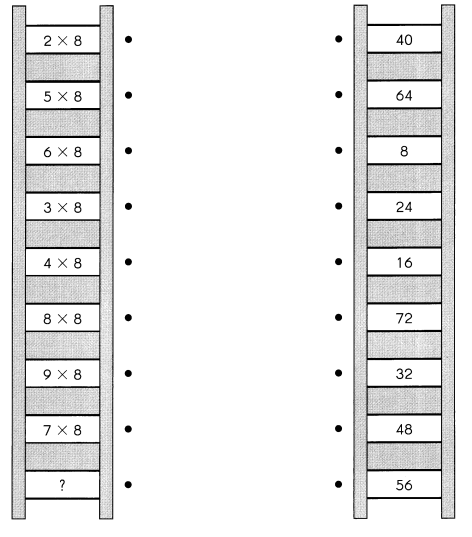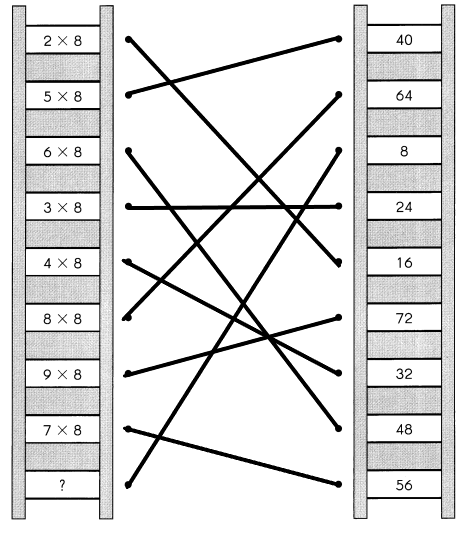Question 13.
What is the missing multiplication fact? ___
8×1 =8.

Explanation:
The missing multiplication fact is 8×1 which is 8.

Solve.

Example
An octagon has 8 equal sides.
How many sides do 5 octagons have?
5 × 8 = 40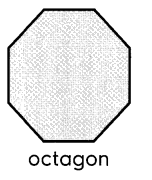5 octagons have 40 sides.

Question 14.
8 children make up a team.
How many children make up 7 teams?
____ children make up 7 teams.
56 children make up 7 teams.

Explanation:
Given that 8 children make up a team. So the number of children makeup 7 teams is
8×7 which is 56 children.

Question 15.
A chocolate cake has 8 cherries.
How many cherries do 6 such cakes have?
6 such cakes have ___ cherries.